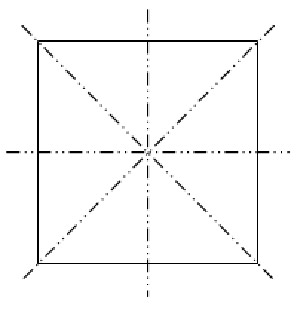A# axis of symmetryAxes of symmetry of a square.

An axis of symmetry is a line drawn through a geometric figure such that the figure is symmetrical (see symmetry) about it. The line may be considered as an axis of rotation: if the figure is rotated about it, there will be two or more positions which are indistinguishable from each other. If the letter Z, for example, is rotated about an axis drawn perpendicularly into the paper through the center of the diagonal stroke, there will be two correspondent positions: the letter has 2-fold rotational symmetry about that axis. In general, if a figure has n correspondent positions on rotation about an axis, it is said to have n-fold symmetry about that axis.